# Doppler effect in light

When there is relative motion between source and observer, there is apparent change in frequency of wave which is called Doppler's effect. In the case of light waves, the Doppler effect can be observed when either the source of light or the observer is moving relative to the other. When the source of light is moving towards the observer, the frequency of the light waves appears to be higher, resulting in a blue shift. Conversely, when the source of light is moving away from the observer, the frequency appears to be lower, resulting in a red shift.

To find Doppler effect in light, consider transformation of Ko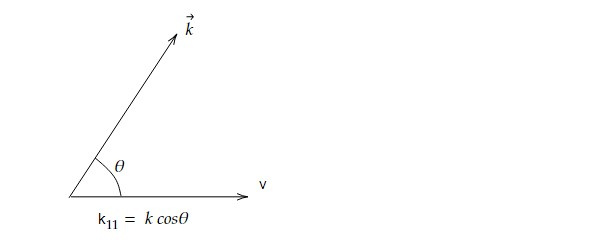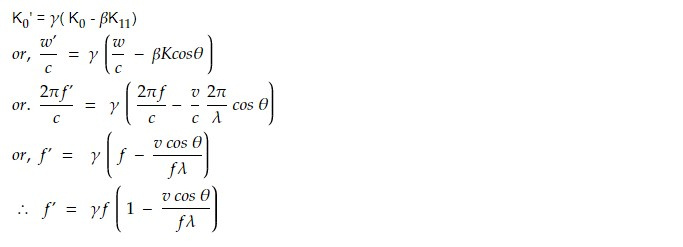Now, velocity of wave = f λ =u

f' = γf ( 1- vcos θ /u)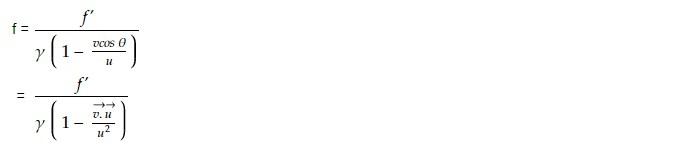This gives us Doppler effect.

Suppose that the source is fixed in S' frame (moving) and observer is fixed in S' frame (rest). Since the source appears to be rest in S', then the frequency f' is called proper frequency.

Let f' = fo then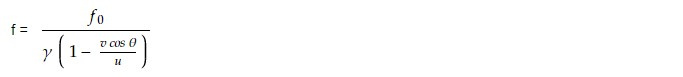Suppose the source is moving away from the observer.

θ = 180°

cosθ = -1

v cosθ = -v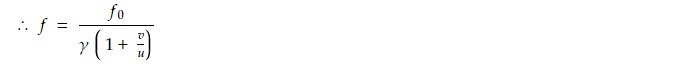In case of light u =c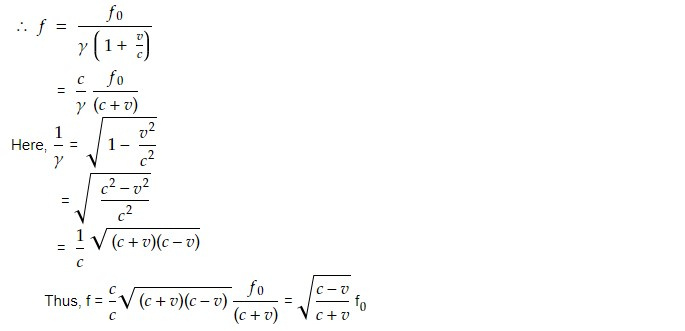Here f is decreasing so λ is increasing. This is known as Red Shift. If the source is moving towards observer.

θ = 0°

cos θ = 1

vcos θ = v

So,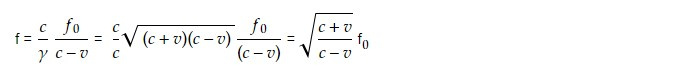Frequency is increasing and λ is decreasing, this is called blue shift.

Taking the square root of this ratio gives the factor by which the wave's frequency changes due to the Doppler effect. The fraction inside the square root represents the ratio of the wave's relative speed when the source is moving towards the observer to the wave's relative speed when the source is moving away from the observer.

The frequency shift of a wave due to the relative motion of the source and the observer, as described by the Doppler effect.

This effect has important implications in astronomy, where it is used to measure the relative motion of celestial objects. By observing the spectrum of light emitted by a celestial object, astronomers can determine whether the object is moving towards or away from Earth, and by how much, based on the degree of shift in the light's frequency.

The Doppler effect has a wide range of applications in various fields, including:

1. Astronomy: The Doppler effect is used in astronomy to measure the relative motion of celestial objects, such as stars and galaxies. By analyzing the spectrum of light emitted by these objects, astronomers can determine whether they are moving towards or away from Earth, and by how much.

2. Radar: Radar systems use the Doppler effect to measure the velocity of moving objects, such as aircraft and ships. By transmitting a radio signal towards the moving object and then analyzing the frequency shift of the reflected signal, the radar system can determine the object's velocity.

3. Medical diagnostics: The Doppler effect is used in medical diagnostics to measure blood flow in the body. This is done by emitting high-frequency sound waves towards a blood vessel and analyzing the frequency shift of the reflected waves, which can provide information on the direction and velocity of blood flow.

4. Weather forecasting: Doppler radar is used in weather forecasting to measure the velocity and direction of precipitation, such as rain or snow. By analyzing the frequency shift of the radar signal reflected by the precipitation, meteorologists can track the movement and intensity of storms.

5. Sound engineering: The Doppler effect is used in sound engineering to simulate the effect of moving sound sources. For example, in video games or movies, the sound of a moving object can be simulated by changing the frequency of the sound wave as the object moves towards or away from the listener.

This note is a part of the Physics Repository.